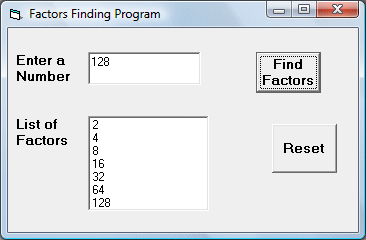# Factors Finder

This is a program that can find factors of a number entered by the user and display them in a list box.  We use the simple logic that a number is divisible by all its factors. However, you need to tell Visual Basic how to identify factors from non factors. In order to do this , we can make use of  the fact that the remainder after a number is divided by its factor is zero. In Visual Basic, you can use the MOD operator, which compute the value of the remainder after a number is divided by an integer. The format is N Mod x.

With these logics in mind, we can use a For....Next Loop to evaluate all the remainders for all the divisors of N smaller than N. If the remainder is zero, then the divisor x is added to the list box. In this way, we are able to compute all the factors.

### The Interface### The code

Private Sub Command1_Click()
Dim N, x As Integer
N = Val(Text1.Text)
For x = 2 To N - 1
If N Mod x = 0 Then